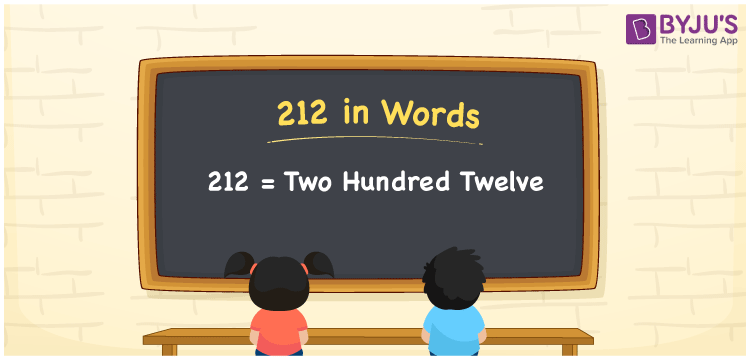# 212 in Words

The number 212 in words is written as Two Hundred Twelve. For example, if you bought a perfume worth Rs. 212, then you can say, “I bought a perfume worth Rupees Two Hundred Twelve”. We know that 212 is a cardinal number since it depicts a certain amount. In this article, we will learn how to spell and write the number 212 in English.

 212 in Words Two Hundred Twelve Two Hundred Twelve in numerical form 212

## 212 in English Words

Generally, we write the numbers in words using the English alphabet. Therefore, we can read the number 212 in English as Two Hundred Twelve.## How to Write 212 in Words?

212 is a three-digit number. Hence, let us create a table of 3 columns. The below table shows the place value chart for the number 212.

 Hundreds Tens Ones 2 1 2

Hence, we can write the expanded form as:

2 x Hundred + 1 x Ten + 2 x One

= 2 x 100 + 1 x 10 + 2 x 1

= 200 + 10 + 2

= 212

= Two Hundred Twelve

Therefore, 212 in words is written as Two Hundred Twelve

Interesting way of writing 212 in words

2 = Two

21 = Twenty-One

212 = Two Hundred Twelve

Thus, the word form of the number 212 is Two Hundred Twelve

212 is a natural number that is the successor of 211 and the predecessor of 213

• 212 in words – Two Hundred Twelve
• Is 212 an odd number? – No
• Is 212 an even number? – Yes
• Is 212 a perfect square number? – No
• Is 212 a perfect cube number? – No
• Is 212 a prime number? – No
• Is 212 a composite number? – Yes

## Frequently Asked Questions on 212 in Words

Q1

### Write 212 in words.

212 in words is written as Two Hundred Twelve.
Q2

### Simplify 100 + 112, and express in words.

Simplifying 100 + 112, we get 212. Thus, 212 in words is written as Two Hundred Twelve.
Q3

### Write Two Hundred Twelve in numbers.

Two Hundred Twelve in numbers is 212.